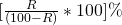# Percentage

To expressas a fraction =Thus,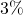==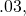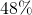=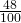==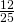To expressas a percentage, we have=Thus=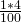== 25%

if the price of commodity increases by R% than the reduction in consumption so as not to increase the expenditure is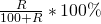Do – decreases R% ______ do__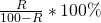Result on Population– let the population of a town be P now & suppose increases at the rate of (@) R % annum then.
Population after n year =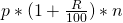Population n year ago =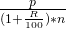Result on depreciation (decrease-) Let the present value of a machine BCp suppose it depreciate @ R% per annum then.
Value of machine after n year =Value of machine n year ago =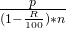if A is R% more than B, B is less than A byIf A is R% less than B, then B is more than A by